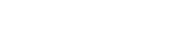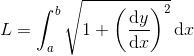# Category: Limits## Arc length formula

Today we are going to find the formula that allows us to calculate the arc length given a function f(x). This is quite intuitive, so you should have no problem!… Read more Arc length formula## Cauchy principal value | Definite integral of 1/x

We know that the integral of 1/x is the natural logarithm ln(x). Very straightforward, yes, but it’s not as simple when it comes to evaluating the definite integral. As you… Read more Cauchy principal value | Definite integral of 1/x

## L’Hôpital’s rule

Evaluating the limit of x-3 with x approaching 4 is very easy and straightforward: you just plug in the value x tends to and you’re done! Another example: How about this? As we saw here, one divided by zero equals infinity, therefore one divided by infinity equals zero. We can’t evaluate limits directly by substitution when, if we do substitute, we get an indeterminate result, like infinity/infinity, 0/0, infinity – infinity, 0 times infinity, infinity/0. When this happens, we need to rewrite the limit, without substitution, and apply L’Hôpital’s rule.… Read more L’Hôpital’s rule

## How to solve definite integrals

Indefinite integrals are used to find the formula for the area under a curve of function f(x), whereas definite integrals allow you to calculate the value of the area. It’s like the area of a rectangle, b*h: this is the formula, and in order to find the value you have to plug in the values of b and h. The same is for definite integrals. A definite integral is generally written like this: where a and b are called respectively lower and upper bounds. Also, As you can see, if… Read more How to solve definite integrals

## Division by zero & what is infinity?

“You can’t divide a number by 0” how many times have you heard this? Don’t you think that even the seemingly impossible can actually be possible? Let’s think of zero as a really small number, like 0.0000000000001; we are going to divide by a much bigger number, like 100, until we get to our new “definition” of zero. Let’s first see what 1 divided by 0 is equal to by using the approach I have just mentioned. 1/100=0.01 1/50=0.02 1/10=0.1 1/5=0.2 1/1=1 1/0.5=2 1/0.1=10 1/0.01=100 1/0.001=1000 1/0.0001=10000 1/0.00001=100000 You can… Read more Division by zero & what is infinity?

{{#pages}} {{/pages}}
%%footer%%